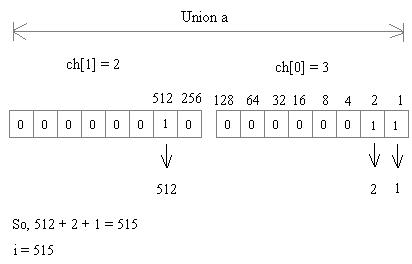# C Programming - Structures, Unions, Enums

Exercise : Structures, Unions, Enums - Find Output of Program
1.
What will be the output of the program ?
``````#include<stdio.h>

int main()
{
union a
{
int i;
char ch;
};
union a u;
u.ch=3;
u.ch=2;
printf("%d, %d, %d\n", u.ch, u.ch, u.i);
return 0;
}
``````
3, 2, 515
515, 2, 3
3, 2, 5
515, 515, 4
Explanation:

The system will allocate 2 bytes for the union.

The statements u.ch=3; u.ch=2; store data in memory as given below.2.
What will be the output of the program ?
``````#include<stdio.h>

int main()
{
union var
{
int a, b;
};
union var v;
v.a=10;
v.b=20;
printf("%d\n", v.a);
return 0;
}
``````
10
20
30
0
Explanation:
No answer description is available. Let's discuss.

3.
What will be the output of the program ?
``````#include<stdio.h>

int main()
{
struct value
{
int bit1:1;
int bit3:4;
int bit4:4;
}bit={1, 2, 13};

printf("%d, %d, %d\n", bit.bit1, bit.bit3, bit.bit4);
return 0;
}
``````
1, 2, 13
1, 4, 4
-1, 2, -3
-1, -2, -13
Explanation:

Note the below statement inside the struct:

int bit1:1; --> 'int' indicates that it is a SIGNED integer.

For signed integers the leftmost bit will be taken for +/- sign.

If you store 1 in 1-bit field:

The left most bit is 1, so the system will treat the value as negative number.

The 2's complement method is used by the system to handle the negative values.

Therefore, the data stored is 1. The 2's complement of 1 is also 1 (negative).

Therefore -1 is printed.

If you store 2 in 4-bits field:

Binary 2: 0010 (left most bit is 0, so system will treat it as positive value)

0010 is 2

Therefore 2 is printed.

If you store 13 in 4-bits field:

Binary 13: 1101 (left most bit is 1, so system will treat it as negative value)

Find 2's complement of 1101:

1's complement of 1101 : 0010
2's complement of 1101 : 0011 (Add 1 to the result of 1's complement)

0011 is 3 (but negative value)

Therefore -3 is printed.

4.
What will be the output of the program in 16 bit platform (Turbo C under DOS) ?
``````#include<stdio.h>

int main()
{
struct value
{
int bit1:1;
int bit3:4;
int bit4:4;
}bit;
printf("%d\n", sizeof(bit));
return 0;
}
``````
1
2
4
9
Explanation:

Since C is a compiler dependent language, in Turbo C (DOS) the output will be 2, but in GCC (Linux) the output will be 4.

5.
What will be the output of the program ?
``````#include<stdio.h>

int main()
{
enum days {MON=-1, TUE, WED=6, THU, FRI, SAT};
printf("%d, %d, %d, %d, %d, %d\n", MON, TUE, WED, THU, FRI, SAT);
return 0;
}
``````
-1, 0, 1, 2, 3, 4
-1, 2, 6, 3, 4, 5
-1, 0, 6, 2, 3, 4
-1, 0, 6, 7, 8, 9